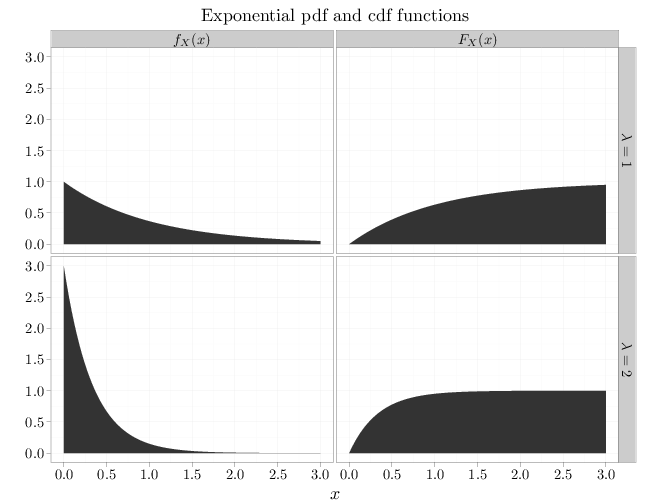## Probability

### The Analysis of Data, volume 1

Important Random Variables: The Exponential Distribution

## 3.8. The Exponential Distribution

The exponential RV, $X\sim \text{Exp}(\lambda)$, where $\lambda > 0$, has the pdf $f_X(x)=\begin{cases}\lambda e^{-\lambda x} & x > 0\\ 0 &\text{otherwise}\end{cases}.$ Since the pdf decreases exponentially as $x$ grows (for positive $x$), it is more probable that $X$ will receive a small positive value than a large positive value. The cdf is $F_X(x)=\P(X\leq x)=\begin{cases} \int_0^x \lambda e^{-\lambda x}=-e^{-\lambda x}\Big|_0^x=1-e^{-\lambda x} & x > 0 \\ 0 & \text{otherwise}\end{cases}.$

The exponential RV is the only continuous RV $X$ with the memoryless property: the probability that $X$ is larger than $s+t$ is the same as the probability that $X$ is larger than $s$ in one experiment and an independent copy of $X$ is larger than $t$ in an independent experiment \begin{align} \P(X > s+t) = \P(X > s)\P(X > t). \end{align} (The equation above holds for the exponential RV since $\P(X > t)=1-F_X(t)=e^{-\lambda x}$.) The term "memoryless" is motivated by noting that $\P( X >s+t) = \P(X > s)\P(X > t)$ implies the following lack of memory: \begin{align*} \P(X > t+h|X > t)&=\frac{\P(\{X > t+h\}\cap\{X > t\})}{\P(X > t)} =\frac{\P(\{X > t+h\})}{\P(X > t)}\\ &=\frac{e^{-\lambda(t+h)}}{e^{-\lambda t}}=e^{-\lambda h}=\P(X > h). \end{align*} A proof that no other continuous distribution has this property is available for example in (Feller, 1968). The memoryless property motivates the use of the exponential RV to model times between successive arrivals of customers at a store, cars at an intersection, or phone calls at a switchboard.

The mgf of an exponential RV is \begin{align*} m(t)&=\E(\exp(tX))=\lambda\int_0^{\infty} e^{-\lambda x} e^{tx}\,dx= \lambda\int_0^{\infty} e^{(t-\lambda)x} \,dx =\frac{\lambda}{t-\lambda} e^{(t-\lambda)x}\Big|_0^{\infty}\\ &=\frac{\lambda}{\lambda-t} \end{align*} for $t<\lambda$, implying that \begin{align*} \E(X)& = m'(0) = \frac{\lambda}{(\lambda-t)^2}\Big|_{t=0} = \lambda^{-1},\\ \Var(X) &= m''(0) = \frac{\lambda}{(\lambda-t)^3}\Big|_{t=0} = \lambda^{-2}. \end{align*}

The R code below graphs the pdf and cdf of exponential RVs with different parameter values.

x = seq(0, 3, length = 100)
y1 = dexp(x, 1)
y2 = dexp(x, 3)
y3 = pexp(x, 1)
y4 = pexp(x, 3)
D = data.frame(probability = c(y1, y2, y3, y4), x = x)
D$parameter[1:100] = "$\\lambda=1$" D$parameter[101:200] = "$\\lambda=2$"
D$parameter[201:300] = "$\\lambda=1$" D$parameter[301:400] = "$\\lambda=2$"
D$type[1:200] = "$f_X(x)$" D$type[201:400] = "$F_X(x)$"
qplot(x, probability, data = D, geom = "area", facets = parameter ~
type, xlab = "$x$", ylab = "", main = "Exponential pdf and cdf functions")